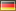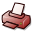# A Fast Matrix-Free Algorithm for Spectral Approximations to High-Dimensional Partial Differential Equations

## DSpace Repository

 Dateien:

 URI: Dokumentart: Dissertation Date: 2016-01 Language: English Faculty: 7 Mathematisch-Naturwissenschaftliche Fakultät7 Mathematisch-Naturwissenschaftliche Fakultät Department: Mathematik Advisor: Lubich, Christian (Prof. Dr.) Day of Oral Examination: 2016-01-13 DDC Classifikation: 510 - Mathematics Keywords: Schrödinger-Gleichung , Spektralmethode , Differentialgleichung Other Keywords:Partielle DifferentialgleichungenQuantendynamikMatrixfreier AlgorithmusSpektralmethodenHochdimensionale GleichungenSchrödingergleichungNumerische MathematikNumerical AnalysisSchrödinger equationHigh-dimensional equationsPartial differential equationsQuantum dynamicsMatrix-free algorithmSpectral methods License: Order a printed copy:Print-on-Demand Show full item record

### Inhaltszusammenfassung:

Das Lösen hochdimensionaler partieller Differentialgleichungen mittels Spektralmethoden stellt in Anbetracht der damit verbundenen enormen Speicherplatz- wie auch Zeitkomplexität eine besondere Herausforderung dar. Wir betrachten das Beispiel der zeitabhäng-igen Vielteilchen-Schrödingergleichung, die mit einem Galerkinansatz im Raum diskretisiert wird. Die zugrundeliegende Basis besteht aus Tensorprodukten von Hermitefunktionen. Bei der Integration des zugehörigen Systems gewöhnlicher Differentialgleichungen sind in jedem Zeitschritt typerischerweise Matrix-Vektor-Produkte mit der Darstellungsmatrix des Hamiltonoperators der Gleichung bezüglich der gewählten Galerkinbasis zu berechnen. Die Größe dieser Matrix hängt im Falle einer nicht ausgedünnten Basis exponentiell von der Dimension des Problems ab. Das explizite Aufstellen der Matrix überschreitet damit leicht den zur Verfügung stehenden Speicherplatz und erfordert unerträglich lange Rechenzeiten. Diese Arbeit stellt einen schnellen Algorithmus zur Berechnung solcher Matrix-Vektor-Produkte vor, dessen Komplexität nur linear von der Größe der Galerkinbasis abhängt und der ohne explizites Aufstellen der Matrix auskommt. Darüber hinaus erlaubt er nahezu beliebiges Ausdünnen der Basis - sofern eine entsprechende Ausdünnung selbst eine gute Approximation an die gesuchte Lösung des Problems liefert. Stellt man die Ortsoperatoren bezüglich der einzelnen Koordinaten in der gewählten Basis dar, so lassen sich mithilfe der wechselseitigen Orthogonalität der Hermitefunktionen sowie der sie definierenden Drei-Term-Rekursion Produkte dieser Koordinatenmatrizen mit Vektoren in linearer Zeit berechnen. Die bereits von E. Faou, V. Gradinaru und Ch. Lubich vorgestellte Kernidee des schnellen Algorithmus besteht nun darin, die Koordinatenmatrizen formal in eine polynomielle Approximation des Potentials einzusetzen. Wir modifizieren diesen Vorschlag und präsentieren einen rigorosen Algorithmus. Für eine nicht ausgedünnte Galerkinbasis erweist sich diese Idee als äquivalent zur Approximation der Integrale in jedem Eintrag der Matrixdarstellung des Potentials mittels einer spezifisch gewählten Gauß-Hermite-Quadratur, wie in der vorliegenden Arbeit gezeigt wird. Ausdünnen der Basis generiert einen zusätzlichen Fehler. Ein wichtiger Bestandteil dieser Arbeit ist die Fehleranalyse. Insbesondere lassen sich die durch Quadratur und ggf. Ausdünnen der Basis verursachten Fehler jeweils durch geschicktes Umschreiben der Hermite-Rekursion als Binärbaum kontrollieren. Unter der Annahme eines im Vergleich zur exakten Lösung hinreichend glatten Potentials fallen diese Fehler spektral ab. Dies wird in numerischen Experimenten bestätigt. Als Vergleichsmaß für die tatsächliche Einsparung an Rechenzeit durch den schnellen Algorithmus dient uns ein matrixfreier Ansatz, der in der chemischen Literatur entwickelt wurde. Darüber hinaus übertragen wir den obigen Ansatz auf eine analoge Behandlung u.a. der nichtlinearen Schrödingergleichung und von Anfangsrandwertproblemen. Als Beispiel für letztere Klasse betrachten wir im zweiten Teil der Arbeit die Wellengleichung mit variablen Koeffizienten und Engquist-Majda-Randbedingungen und konstruieren analoge effiziente Verfahren für die zugehörigen Matrix-Vektor-Produkte. Wir führen ebenfalls eine Fehleranalyse durch und präsentieren numerische Experimente.

### Abstract:

This thesis is concerned with the computational intractabilities that arise from spectral discretizations of high-dimensional partial differential equations. Using the example of the time-dependent multi-particle Schrödinger equation, we consider a spectral Galerin approximation in space with a tensor product basis of Hermite functions. When propagating the resulting system of ordinary differential equations in time, one typically needs to evaluate matrix-vector products involving a matrix representation of the Hamiltonian operator in each time step. Since the size of this matrix equals the number of equations, which (for an unreduced basis) depends exponentially on the dimension and thus quickly becomes enormously large, this can make computations infeasible - both due to a lack of memory and due to unbearably long computation times. We present a fast algorithm for an efficient computation of these matrix-vector products that scales only linearly with the size of the Galerkin basis - without assembling the matrix itself. Besides being a matrix-free approach, the fast algorithm is compatible with the idea of reducing the index set that underlies the basis. The computational speed-up is achieved using orthogonality of the Hermite functions in combination with their generic three-term recurrence. Briefly, these properties allow to compute the action of the matrix representations of the coordinatewise position operators on vectors in linear time. The basic idea is then to insert these coordinate matrices into a polynomial approximate of the potential. This has originally been proposed by E. Faou, V. Gradinaru, and Ch. Lubich. We modify their approach and turn it into a rigorous algorithm. For an unreduced set of basis functions, we show this tentative proceeding to be equivalent to a suitable entrywise approximation of the potential matrix by Gauß-Hermite quadrature. Reducing the index set for the basis functions yields an additional error. We derive error estimates for all approximation steps involved. In particular, using a binary tree approach, bounds for the errors due to quadrature and index set reduction are deduced. Both errors decay spectrally if the potential is significantly smoother than the exact solution wherever the latter does not essentially vanish. Extensive numerical experiments corroborate these findings. Besides, we show performance tests comparing the fast algorithm to a matrix-free approach from the chemical literature. Apart from the above basic form of the fast algorithm, we present applications of the general methodology to the nonlinear Schrödinger equation and, most prominently, to initial-boundary value problems. As an example, we study the acoustic wave equation with non-constant coefficients and Engquist-Majda boundary conditions, and construct efficient procedures for the different kinds of matrix-vector products together with an error analysis and numerical tests.